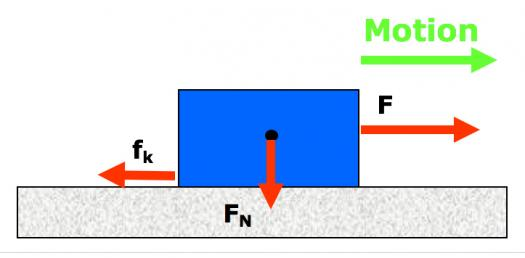# Friction History Trivia Facts! Quiz

10 Questions | Total Attempts: 159SettingsFriction is important because thanks to it the first humans were able to figure out how to make fire. It is also important to study it for disciplines like construction and even sciences that depend on o studying how liquid bodies can be used to measure things like temperature, for instance. So, what do you know about the history of friction? Take our quiz and find out now.

Related Topics
• 1.
Who were the first ones to study friction?
• A.

The Egyptians

• B.

The Germans

• C.

The Greeks

• D.

The Romans

• 2.
Who discovered the classic laws of sliding friction?
• A.

Leonardo Da Vinci

• B.

Magelan

• C.

Isaac Newton

• D.

Nicola Tesla

• 3.
When were the classic laws of sliding discovered?
• A.

In 1493

• B.

In 1495

• C.

In 1496

• D.

In 1499

• 4.
Who developed the understanding of friction in 1785?
• A.

Thomas Edison

• B.

Pascale

• C.

Galileo

• D.

Charles -Augustin de Coulomb

• 5.
How many factors on friction did Coulomb investigate on?
• A.

4

• B.

5

• C.

6

• D.

3

• 6.
What was the focus of research of friction during the 20th Century?
• A.

To understand the mechanisms behind friction

• B.

To understand the physical mechanisms behind friction

• C.

To understand the material mechanisms behind friction

• D.

To understand the invisible mechanisms behind friction

• 7.
When was the atomic force microscope created?
• A.

In 1977

• B.

In 1979

• C.

In 1986

• D.

In 1982

• 8.
What is known as mechanical energy?
• A.

It's the sum of potential energy and kinetic energy

• B.

It's electrical energy

• C.

It's water generated energy

• D.

It's solar energy

• 9.
What's the nature of friction?
• A.

It's a powerful force

• B.

It's a non conservative force

• C.

It's something you can observe

• D.

It's a non static force

• 10.
How many types of friction are there?
• A.

3

• B.

4

• C.

5

• D.

6